Anúncio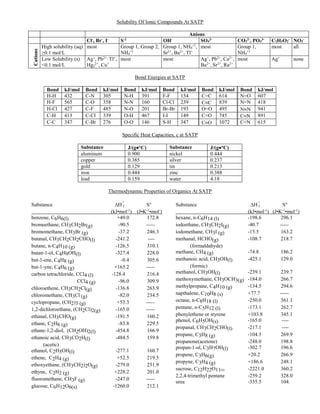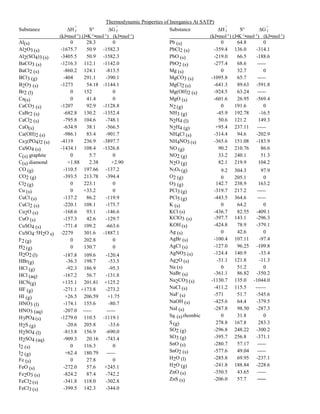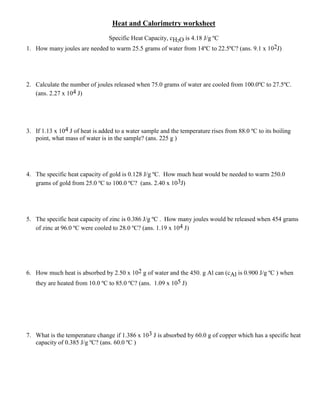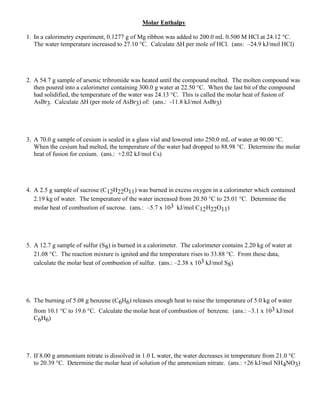Anúncio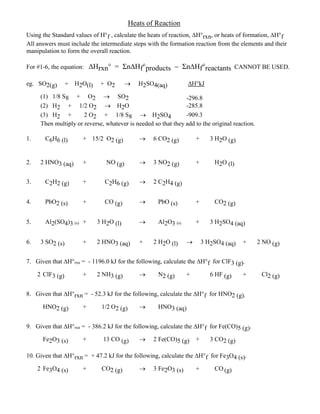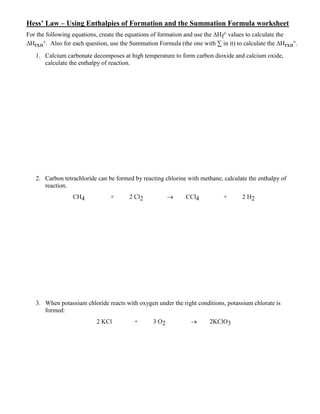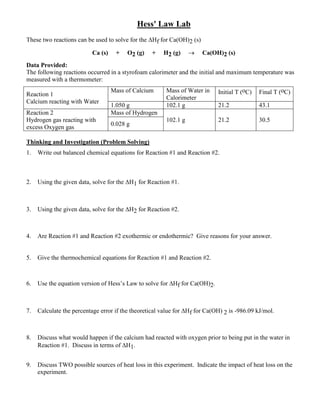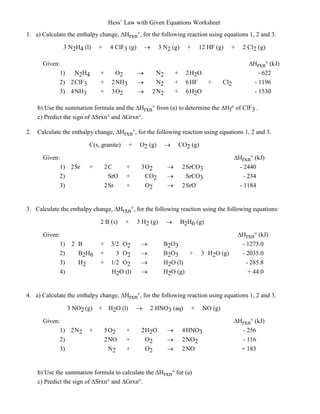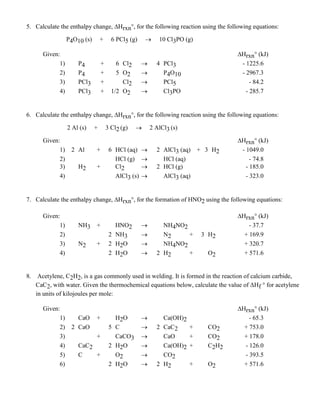Anúncio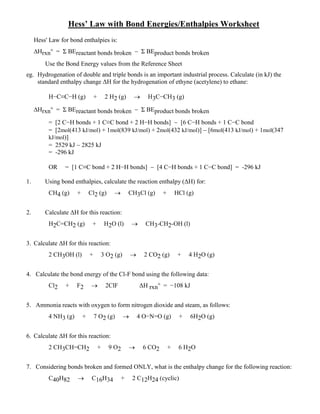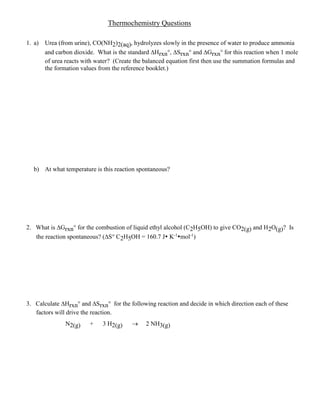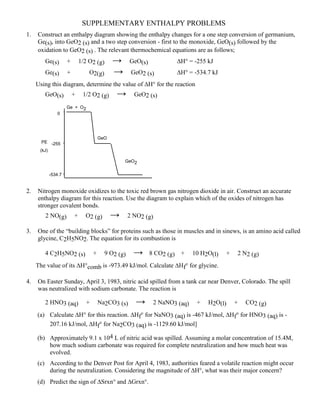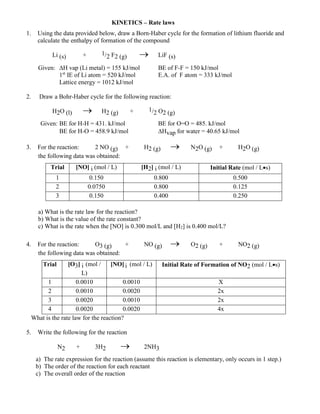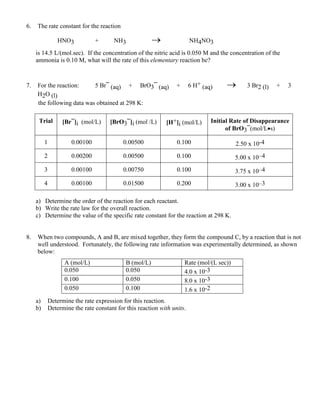Anúncio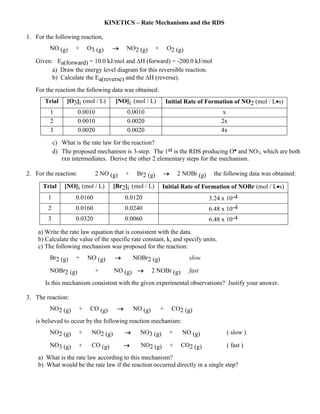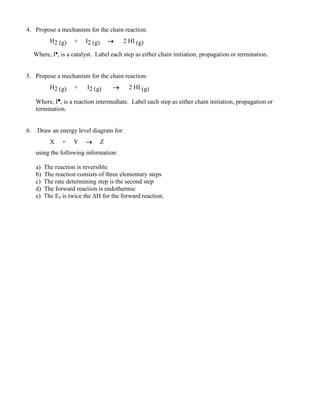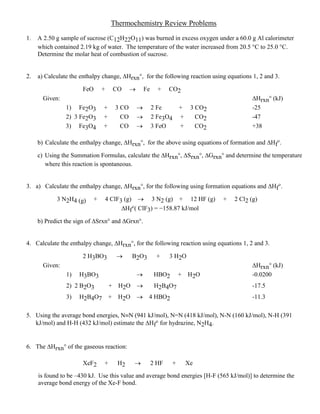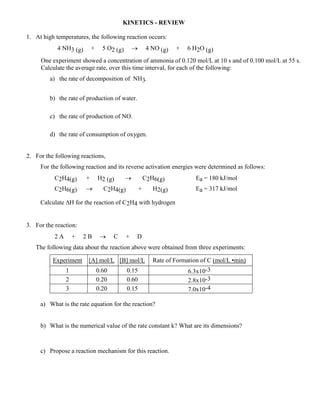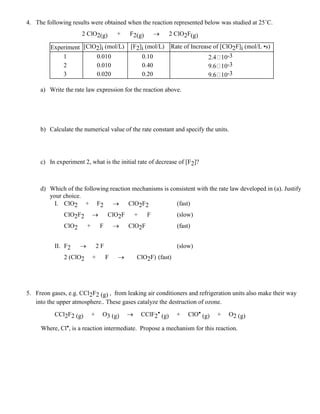Anúncio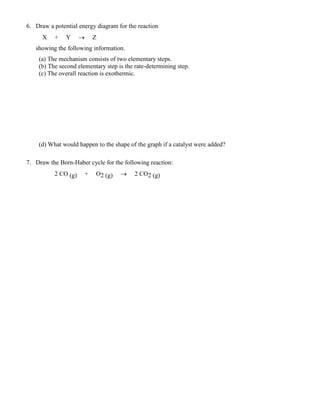Próximos SlideShares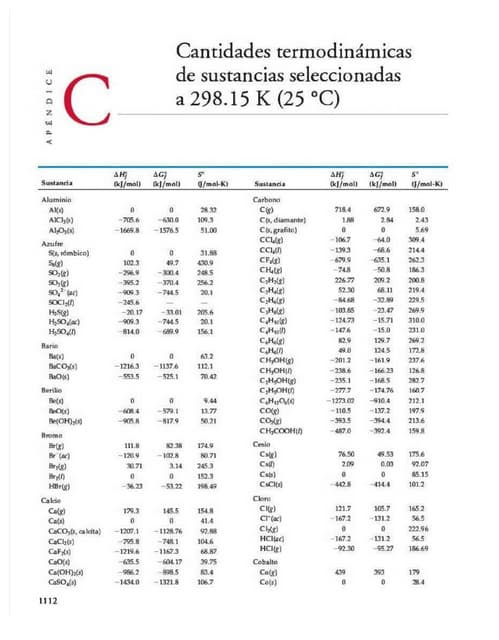Pdf tabla-de-entalpias-energia-libre-de-gibbs-y-entropia compress
Carregando em ... 3
1 de 20
Anúncio

### Thermo kin ws complete

1. Solubility Of Ionic Compounds At SATP Anions Cl- , Br- , I- S-2 OH- SO4 2- CO3 2- , PO4 3- C2H3O2 - NO3 - Cations High solubility (aq) ≥0.1 mol/L most Group 1, Group 2, NH4 +1 Group 1, NH4 +1 , Sr2+ , Ba2+ , Tl+ most Group 1, NH4 +1 most all Low Solubility (s) <0.1 mol/L Ag+ , Pb2+, Tl+ , Hg2 2+ , Cu+ most most Ag+ , Pb2+ , Ca2+ , Ba2+ , Sr2+ , Ra2+ most Ag+ none Bond Energies at SATP Bond kJ/mol Bond kJ/mol Bond kJ/mol Bond kJ/mol Bond kJ/mol Bond kJ/mol H-H 432 C-N 305 N-H 391 F-F 154 C=C 614 N=O 607 H-F 565 C-O 358 N-N 160 Cl-Cl 239 CC 839 N=N 418 H-Cl 427 C-F 485 N-O 201 Br-Br 193 O=O 495 NN 941 C-H 413 C-Cl 339 O-H 467 I-I 149 C=O 745 CN 891 C-C 347 C-Br 276 O-O 146 S-H 347 CO 1072 C=N 615 Specific Heat Capacities, c at SATP Substance J/(g°C) Substance J/(g°C) aluminum 0.900 nickel 0.444 copper 0.385 silver 0.237 gold 0.129 tin 0.213 iron 0.444 zinc 0.388 lead 0.159 water 4.18 Thermodynamic Properties of Organics At SATP Substance ∆H f ° S° (kJ•mol-1 ) (J•K-1 •mol- ) benzene, C6H6(l) +49.0 172.8 bromoethane, CH3CH2Br(g) -90.5 ----- bromomethane, CH3Br (g) -37.2 246.3 butanal, CH3CH2CH2CHO(l) -241.2 ---- butane, n-C4H10 (g) -126.5 310.1 butan-1-ol, C4H9OH(l) -327.4 228.0 but-1-ene, C4H8 (g) -0.4 305.6 but-1-yne, C4H6 (g) +165.2 ----- carbon tetrachloride, CCl4 (l) -128.4 216.4 CCl4 (g) -96.0 309.9 chloroethene, CH3CH2Cl(g) -136.8 263.9 chloromethane, CH3Cl (g) -82.0 234.5 cyclopropane, (CH2)3 (g) +53.3 ----- 1,2-dichloroethane, (CH2Cl)2(g) -165.0 ----- ethanal, CH3CHO(g) -191.5 160.2 ethane, C2H6 (g) -83.8 229.5 ethane-1,2-diol, (CH2OH)2(l) -454.8 166.9 ethanoic acid, CH3CO2H(l) -484.5 159.8 (acetic) ethanol, C2H5OH(l) -277.1 160.7 ethene, C2H4 (g) +52.5 219.5 ethoxyethane, (CH3CH2)2O(g) -279.0 251.9 ethyne, C2H2 (g) +228.2 201.0 fluoromethane, CH3F (g) -247.0 ----- glucose, C6H12O6(s) -1260.0 212.1 Substance ∆H f ° S° (kJ•mol-1 ) (J•K-1 •mol-1 ) hexane, n-C6H14 (l) -198.6 296.1 iodoethane, CH3CH2I(g) -40.7 ----- iodomethane, CH3I (g) -15.5 163.2 methanal, HCHO(g) -108.7 218.7 (formaldahyde) methane, CH4 (g) -74.8 186.2 methanoic acid, CH3OH(l) -425.1 129.0 (formic) methanol, CH3OH(l) -239.1 239.7 methoxymethane, CH3OCH3(g) -184.0 266.7 methylpropane, C4H10 (g) -134.5 294.6 napthalene, C10H8 (s) +77.7 ----- octane, n-C8H18 (l) -250.0 361.1 pentane, n-C5H12 (l) -173.1 262.7 phenylethene or styrene +103.8 345.1 phenol, C6H5OH(s) -165.0 ---- propanal, CH3CH2CHO(l) -217.1 ---- propane, C3H8 (g) -104.5 269.9 propanone(acetone) -248.0 198.8 propan-1-ol, C3H7OH(l) -302.7 196.6 propene, C3H6(g) +20.2 266.9 propyne, C3H4 (g) +186.6 248.1 sucrose, C12H22O11(s) -2221.0 360.2 2,2,4-trimethyl pentane -259.2 328.0 urea -335.5 104.
2. Thermodynamic Properties of Inorganics At SATP) Substance ∆H f ° S° ∆G f ° (kJ•mol-1 ) (J•K-1 •mol-1 ) (kJ•mol-1 ) Al(s) 0 28.3 0 Al2O3 (s) -1675.7 50.9 -1582.3 Al2(SO4)3 (s) -3405.5 50.9 -1582.3 BaCO3 (s) -1216.3 112.1 -1142.0 BaCl2 (s) -860.2 124.1 -813.5 BCl3 (g) -404 291.1 -390.1 B2O3 (s) -1273 54.18 -1144.1 Br2 (l) 0 152 0 Ca(s) 0 41.4 0 CaCO3 (s) -1207 92.9 -1128.8 CaBr2 (s) -682.8 130.2 -1352.4 CaCl2 (s) -795.8 104.6 -748.1 CaO(s) -634.9 38.1 -566.5 Ca(OH)2 (s) -986.1 83.4 -901.7 Ca3(PO4)2 (s) -4119 236.9 -3897.7 CaSO4 (s) -1434.1 108.4 -1326.8 C(s) graphite 0 5.7 0 C(s) diamond +1.88 2.38 +2.90 CO (g) -110.5 197.66 -137.2 CO2 (g) -393.5 213.78 -394.4 Cl2 (g) 0 223.1 0 Cu (s) 0 +33.2 0 CuCl (s) -137.2 86.2 -119.9 CuCl2 (s) -220.1 108.1 -175.7 Cu2O (s) -168.6 93.1 -146.6 CuO (s) -157.3 42.6 -129.7 CuSO4 (s) -771.4 109.2 -663.6 CuSO4·5H2O s) -2279 301.6 -1887.1 F2 (g) 0 202.8 0 H2 (g) 0 130.7 0 H2O2 (l) -187.8 109.6 -120.4 HBr(g) -36.3 198.7 -53.5 HCl (g) -92.3 186.9 -95.3 HCl (aq) -167.2 56.7 -131.8 HCN(g) +135.1 201.81 +125.2 HF (g) -271.1 +173.8 -273.2 HI (g) +26.5 206.59 +1.75 HNO3 (l) -174.1 155.6 -80.7 HNO3 (aq) -207.0 ----- ----- H3PO4 (s) -1279.0 110.5 -1119.1 H2S (g) -20.6 205.8 -33.6 H2SO4 (l) -813.8 156.9 -690.0 H2SO4 (aq) -909.3 20.16 -743.4 I2 (s) 0 116.3 0 I2 (g) +62.4 180.79 ----- Fe (s) 0 27.8 0 FeO (s) -272.0 57.6 +245.1 Fe2O3 (s) -824.2 87.4 -742.2 FeCl2 (s) -341.8 118.0 -302.8 FeCl3 (s) -399.5 142.3 -344.0 Substance ∆H f ° S° ∆G f ° (kJ•mol-1 ) (J•K-1 •mol-1 ) (kJ•mol-1 ) Pb (s) 0 64.8 0 PbCl2 (s) -359.4 136.0 -314.1 PbO (s) -219.0 66.5 -188.6 PbO2 (s) -277.4 68.6 ----- Mg (s) 0 32.7 0 MgCO3 (s) -1095.8 65.7 ----- MgCl2 (s) -641.3 89.63 -591.8 Mg(OH)2 (s) -924.5 63.24 ----- MgO (s) -601.6 26.95 -569.4 N2 (g) 0 191.6 0 NH3 (g) -45.9 192.78 -16.5 N2H4 (l) 50.6 121.2 149.3 N2H4 (g) +95.4 237.11 ----- NH4Cl (s) -314.4 94.6 -202.9 NH4NO3 (s) -365.6 151.08 -183.9 NO (g) 90.2 210.76 86.6 NO2 (g) 33.2 240.1 51.3 N2O (g) 82.1 219.9 104.2 N2O4 (g) 9.2 304.3 97.9 O2 (g) 0 205.1 0 O3 (g) 142.7 238.9 163.2 PCl3 (g) -319.7 217.2 ----- PCl5 (g) -443.5 364.6 ----- K (s) 0 64.2 0 KCl (s) -436.7 82.55 -409.1 KClO3 (s) -397.7 143.1 -296.3 KOH (s) -424.8 78.9 -379.1 Ag (s) 0 42.6 0 AgBr (s) -100.4 107.11 -97.4 AgCl (s) -127.0 96.25 -109.8 AgNO3 (s) -124.4 140.9 -33.4 Ag2O (s) -31.1 121.8 -11.3 Na (s) 0 51.2 0 NaBr (s) -361.1 86.82 -350.2 Na2CO3 (s) -1130.7 135.0 -1044.0 NaCl (s) -411.2 115.5 ------ NaF (s) -571 51.7 -545.6 NaOH (s) -425.6 64.4 -379.5 NaI (s) -287.8 98.50 -287.3 S8 (s) rhombic 0 31.8 0 S (g) 278.8 167.8 283.3 SO2 (g) -296.8 248.22 -300.2 SO3 (g) -395.7 256.8 -371.1 SnO (s) -280.7 57.17 ----- SnO2 (s) -577.6 49.04 ----- H2O (l) -285.8 69.95 -237.1 H2O (g) -241.8 188.84 -228.6 ZnO (s) -350.5 43.65 ----- ZnS (s) -206.0 57.7 -----
3. Heat and Calorimetry worksheet Specific Heat Capacity, cH2O is 4.18 J/g ºC 1. How many joules are needed to warm 25.5 grams of water from 14ºC to 22.5ºC? (ans. 9.1 x 102J) 2. Calculate the number of joules released when 75.0 grams of water are cooled from 100.0ºC to 27.5ºC. (ans. 2.27 x 104 J) 3. If 1.13 x 104 J of heat is added to a water sample and the temperature rises from 88.0 ºC to its boiling point, what mass of water is in the sample? (ans. 225 g ) 4. The specific heat capacity of gold is 0.128 J/g ºC. How much heat would be needed to warm 250.0 grams of gold from 25.0 ºC to 100.0 ºC? (ans. 2.40 x 103J) 5. The specific heat capacity of zinc is 0.386 J/g ºC . How many joules would be released when 454 grams of zinc at 96.0 ºC were cooled to 28.0 ºC? (ans. 1.19 x 104 J) 6. How much heat is absorbed by 2.50 x 102 g of water and the 450. g Al can (cAl is 0.900 J/g ºC ) when they are heated from 10.0 ºC to 85.0 ºC? (ans. 1.09 x 105 J) 7. What is the temperature change if 1.386 x 103 J is absorbed by 60.0 g of copper which has a specific heat capacity of 0.385 J/g ºC? (ans. 60.0 ºC )
4. Molar Enthalpy 1. In a calorimetry experiment, 0.1277 g of Mg ribbon was added to 200.0 mL 0.500 M HCl at 24.12 °C. The water temperature increased to 27.10 °C. Calculate ∆H per mole of HCl. (ans: –24.9 kJ/mol HCl) 2. A 54.7 g sample of arsenic tribromide was heated until the compound melted. The molten compound was then poured into a calorimeter containing 300.0 g water at 22.50 °C. When the last bit of the compound had solidified, the temperature of the water was 24.13 °C. This is called the molar heat of fusion of AsBr3. Calculate ∆H (per mole of AsBr3) of: (ans.: -11.8 kJ/mol AsBr3) 3. A 70.0 g sample of cesium is sealed in a glass vial and lowered into 250.0 mL of water at 90.00 °C. When the cesium had melted, the temperature of the water had dropped to 88.98 °C. Determine the molar heat of fusion for cesium. (ans.: +2.02 kJ/mol Cs) 4. A 2.5 g sample of sucrose (C12H22O11) was burned in excess oxygen in a calorimeter which contained 2.19 kg of water. The temperature of the water increased from 20.50 °C to 25.01 °C. Determine the molar heat of combustion of sucrose. (ans.: –5.7 x 103 kJ/mol C12H22O11) 5. A 12.7 g sample of sulfur (S8) is burned in a calorimeter. The calorimeter contains 2.20 kg of water at 21.08 °C. The reaction mixture is ignited and the temperature rises to 33.88 °C. From these data, calculate the molar heat of combustion of sulfur. (ans.: –2.38 x 103 kJ/mol S8) 6. The burning of 5.08 g benzene (C6H6) releases enough heat to raise the temperature of 5.0 kg of water from 10.1 °C to 19.6 °C. Calculate the molar heat of combustion of benzene. (ans.: –3.1 x 103 kJ/mol C6H6) 7. If 8.00 g ammonium nitrate is dissolved in 1.0 L water, the water decreases in temperature from 21.0 °C to 20.39 °C. Determine the molar heat of solution of the ammonium nitrate. (ans.: +26 kJ/mol NH4NO3)
5. Heats of Reaction Using the Standard values of f , calculate the heats of reaction, rxn, or heats of formation, f All answers must include the intermediate steps with the formation reaction from the elements and their manipulation to form the overall reaction. For #1-6, the equation: ∆Hrxn° = Σn∆Hf°products  Σn∆Hf°reactants CANNOT BE USED. eg. SO2(g) + H2O(l) + O2  H2SO4(aq) ∆H°kJ (1) 1/8 S8 + O2  SO2 -296.8 (2) H2 + 1/2 O2  H2O -285.8 (3) H2 + 2 O2 + 1/8 S8  H2SO4 -909.3 Then multiply or reverse, whatever is needed so that they add to the original reaction. 1. C6H6 (l) + 15/2 O2 (g)  6 CO2 (g) + 3 H2O (g) 2. 2 HNO3 (aq) + NO (g)  3 NO2 (g) + H2O (l) 3. C2H2 (g) + C2H6 (g)  2 C2H4 (g) 4. PbO2 (s) + CO (g)  PbO (s) + CO2 (g) 5. Al2(SO4)3 (s) + 3 H2O (l)  Al2O3 (s) + 3 H2SO4 (aq) 6. 3 SO2 (s) + 2 HNO3 (aq) + 2 H2O (l)  3 H2SO4 (aq) + 2 NO (g) 7. Given that ∆H°rxn = - 1196.0 kJ for the following, calculate the ∆H°f for ClF3 (g). 2 ClF3 (g) + 2 NH3 (g)  N2 (g) + 6 HF (g) + Cl2 (g) 8. Given that ∆H°rxn = - 52.3 kJ for the following, calculate the ∆H°f for HNO2 (g). HNO2 (g) + 1/2 O2 (g)  HNO3 (aq) 9. Given that ∆H°rxn = - 386.2 kJ for the following, calculate the ∆H°f for Fe(CO)5 (g). Fe2O3 (s) + 13 CO (g)  2 Fe(CO)5 (g) + 3 CO2 (g) 10. Given that ∆Hrxn = + 47.2 kJ for the following, calculate the ∆H°f for Fe3O4 (s). 2 Fe3O4 (s) + CO2 (g)  3 Fe2O3 (s) + CO (g)
6. Hess’ Law – Using Enthalpies of Formation and the Summation Formula worksheet For the following equations, create the equations of formation and use the ∆Hf° values to calculate the ∆Hrxn°. Also for each question, use the Summation Formula (the one with ∑ in it) to calculate the ∆Hrxn°. 1. Calcium carbonate decomposes at high temperature to form carbon dioxide and calcium oxide, calculate the enthalpy of reaction. 2. Carbon tetrachloride can be formed by reacting chlorine with methane, calculate the enthalpy of reaction. CH4 + 2 Cl2  CCl4 + 2 H2 3. When potassium chloride reacts with oxygen under the right conditions, potassium chlorate is formed: 2 KCl + 3 O2  2KClO3
7. Hess' Law Lab These two reactions can be used to solve for the Hf for Ca(OH)2 (s) Ca (s) + O2 (g) + H2 (g)  Ca(OH)2 (s) Data Provided: The following reactions occurred in a styrofoam calorimeter and the initial and maximum temperature was measured with a thermometer: Reaction 1 Calcium reacting with Water Mass of Calcium Mass of Water in Calorimeter Initial T (oC) Final T (oC) 1.050 g 102.1 g 21.2 43.1 Reaction 2 Hydrogen gas reacting with excess Oxygen gas Mass of Hydrogen 102.1 g 21.2 30.5 0.028 g Thinking and Investigation (Problem Solving) 1. Write out balanced chemical equations for Reaction #1 and Reaction #2. 2. Using the given data, solve for the H1 for Reaction #1. 3. Using the given data, solve for the H2 for Reaction #2. 4. Are Reaction #1 and Reaction #2 exothermic or endothermic? Give reasons for your answer. 5. Give the thermochemical equations for Reaction #1 and Reaction #2. 6. Use the equation version of Hess’s Law to solve for Hf for Ca(OH)2. 7. Calculate the percentage error if the theoretical value for Hf for Ca(OH) 2 is -986.09 kJ/mol. 8. Discuss what would happen if the calcium had reacted with oxygen prior to being put in the water in Reaction #1. Discuss in terms of H1. 9. Discuss TWO possible sources of heat loss in this experiment. Indicate the impact of heat loss on the experiment.
8. Hess’ Law with Given Equations Worksheet 1. a) Calculate the enthalpy change, ∆Hrxn, for the following reaction using equations 1, 2 and 3. 3 N2H4 (l) + 4 ClF3 (g)  3 N2 (g) + 12 HF (g) + 2 Cl2 (g) Given: Hrxn (kJ) 1) N2H4 + O2  N2 + 2H2O - 622 2) 2ClF3 + 2NH3  N2 + 6HF + Cl2 - 1196 3) 4NH3 + 3O2  2N2 + 6H2O - 1530 b) Use the summation formula and the Hrxn from (a) to determine the Hf of ClF3 . c) Predict the sign of ∆Srxn and ∆Grxn. 2. Calculate the enthalpy change, Hrxn, for the following reaction using equations 1, 2 and 3. C(s, granite) + O2 (g)  CO2 (g) Given: Hrxn (kJ) 1) 2Sr + 2C + 3O2  2SrCO3 - 2440 2) SrO + CO2  SrCO3 - 234 3) 2Sr + O2  2SrO - 1184 3. Calculate the enthalpy change, Hrxn, for the following reaction using the following equations: 2 B (s) + 3 H2 (g)  B2H6 (g) Given: Hrxn (kJ) 1) 2 B + 3/2 O2  B2O3 - 1273.0 2) B2H6 + 3 O2  B2O3 + 3 H2O (g) - 2035.0 3) H2 + 1/2 O2  H2O (l) - 285.8 4) H2O (l)  H2O (g) + 44.0 4. a) Calculate the enthalpy change, Hrxn, for the following reaction using equations 1, 2 and 3. 3 NO2 (g) + H2O (l)  2 HNO3 (aq) + NO (g) Given: Hrxn (kJ) 1) 2N2 + 5O2 + 2H2O  4HNO3 - 256 2) 2NO + O2  2NO2 - 116 3) N2 + O2  2NO + 183 b) Use the summation formula to calculate the Hrxn for (a) c) Predict the sign of ∆Srxn and ∆Grxn.
9. 5. Calculate the enthalpy change, Hrxn, for the following reaction using the following equations: P4O10 (s) + 6 PCl5 (g)  10 Cl3PO (g) Given: Hrxn (kJ) 1) P4 + 6 Cl2  4 PCl3 - 1225.6 2) P4 + 5 O2  P4O10 - 2967.3 3) PCl3 + Cl2  PCl5 - 84.2 4) PCl3 + 1/2 O2  Cl3PO - 285.7 6. Calculate the enthalpy change, Hrxn, for the following reaction using the following equations: 2 Al (s) + 3 Cl2 (g)  2 AlCl3 (s) Given: Hrxn (kJ) 1) 2 Al + 6 HCl (aq)  2 AlCl3 (aq) + 3 H2 - 1049.0 2) HCl (g)  HCl (aq) - 74.8 3) H2 + Cl2  2 HCl (g) - 185.0 4) AlCl3 (s)  AlCl3 (aq) - 323.0 7. Calculate the enthalpy change, Hrxn, for the formation of HNO2 using the following equations: Given: Hrxn (kJ) 1) NH3 + HNO2  NH4NO2 - 37.7 2) 2 NH3  N2 + 3 H2 + 169.9 3) N2 + 2 H2O  NH4NO2 + 320.7 4) 2 H2O  2 H2 + O2 + 571.6 8. Acetylene, C2H2, is a gas commonly used in welding. It is formed in the reaction of calcium carbide, CaC2, with water. Given the thermochemical equations below, calculate the value of ΔHf ° for acetylene in units of kilojoules per mole: Given: Hrxn (kJ) 1) CaO + H2O  Ca(OH)2 - 65.3 2) 2 CaO 5 C  2 CaC2 + CO2 + 753.0 3) + CaCO3  CaO + CO2 + 178.0 4) CaC2 2 H2O  Ca(OH)2 + C2H2 - 126.0 5) C + O2  CO2 - 393.5 6) 2 H2O  2 H2 + O2 + 571.6
10. Hess’ Law with Bond Energies/Enthalpies Worksheet Hess' Law for bond enthalpies is: Hrxn = Σ BEreactant bonds broken  Σ BEproduct bonds broken Use the Bond Energy values from the Reference Sheet eg. Hydrogenation of double and triple bonds is an important industrial process. Calculate (in kJ) the standard enthalpy change ΔH for the hydrogenation of ethyne (acetylene) to ethane: H−C≡C−H (g) + 2 H2 (g)  H3C−CH3 (g) Hrxn = Σ BEreactant bonds broken  Σ BEproduct bonds broken = [2 C−H bonds + 1 C≡C bond + 2 H−H bonds]  [6 C−H bonds + 1 C−C bond = [2mol(413 kJ/mol) + 1mol(839 kJ/mol) + 2mol(432 kJ/mol)]  [6mol(413 kJ/mol) + 1mol(347 kJ/mol)] = 2529 kJ  2825 kJ = -296 kJ OR = [1 C≡C bond + 2 H−H bonds]  [4 C−H bonds + 1 C−C bond] = -296 kJ 1. Using bond enthalpies, calculate the reaction enthalpy (ΔH) for: CH4 (g) + Cl2 (g)  CH3Cl (g) + HCl (g) 2. Calculate ΔH for this reaction: H2C=CH2 (g) + H2O (l)  CH3-CH2-OH (l) 3. Calculate ΔH for this reaction: 2 CH3OH (l) + 3 O2 (g)  2 CO2 (g) + 4 H2O (g) 4. Calculate the bond energy of the Cl-F bond using the following data: Cl2 + F2  2ClF ΔH rxn = −108 kJ 5. Ammonia reacts with oxygen to form nitrogen dioxide and steam, as follows: 4 NH3 (g) + 7 O2 (g)  4 O−N=O (g) + 6H2O (g) 6. Calculate ΔH for this reaction: 2 CH3CH=CH2 + 9 O2  6 CO2 + 6 H2O 7. Considering bonds broken and formed ONLY, what is the enthalpy change for the following reaction: C40H82  C16H34 + 2 C12H24 (cyclic)
11. Thermochemistry Questions 1. a) Urea (from urine), CO(NH2)2(aq), hydrolyzes slowly in the presence of water to produce ammonia and carbon dioxide. What is the standard ∆Hrxn, ∆Srxn and ∆Grxn for this reaction when 1 mole of urea reacts with water? (Create the balanced equation first then use the summation formulas and the formation values from the reference booklet.) b) At what temperature is this reaction spontaneous? 2. What is ∆Grxn for the combustion of liquid ethyl alcohol (C2H5OH) to give CO2(g) and H2O(g)? Is the reaction spontaneous? (∆S C2H5OH = 160.7 J K-1 mol-1 ) 3. Calculate ∆Hrxn and ∆Srxn for the following reaction and decide in which direction each of these factors will drive the reaction. N2(g) + 3 H2(g)  2 NH3(g)
12. PE (kJ) 0 Ge + O2 GeO -255 -534.7 GeO2 SUPPLEMENTARY ENTHALPY PROBLEMS 1. Construct an enthalpy diagram showing the enthalpy changes for a one step conversion of germanium, Ge(s), into GeO2 (s) and a two step conversion - first to the monoxide, GeO(s) followed by the oxidation to GeO2 (s) . The relevant thermochemical equations are as follows; Ge(s) + 1/2 O2 (g) → GeO(s) ∆H° = -255 kJ Ge(s) + O2(g) → GeO2 (s) ∆H° = -534.7 kJ Using this diagram, determine the value of ∆H° for the reaction GeO(s) + 1/2 O2 (g) → GeO2 (s) 2. Nitrogen monoxide oxidizes to the toxic red brown gas nitrogen dioxide in air. Construct an accurate enthalpy diagram for this reaction. Use the diagram to explain which of the oxides of nitrogen has stronger covalent bonds. 2 NO(g) + O2 (g) → 2 NO2 (g) 3. One of the “building blocks” for proteins such as those in muscles and in sinews, is an amino acid called glycine, C2H5NO2. The equation for its combustion is 4 C2H5NO2 (s) + 9 O2 (g) → 8 CO2 (g) + 10 H2O(l) + 2 N2 (g) The value of its ∆Hcomb is -973.49 kJ/mol. Calculate ∆Hf for glycine. 4. On Easter Sunday, April 3, 1983, nitric acid spilled from a tank car near Denver, Colorado. The spill was neutralized with sodium carbonate. The reaction is 2 HNO3 (aq) + Na2CO3 (s) → 2 NaNO3 (aq) + H2O(l) + CO2 (g) (a) Calculate ∆H° for this reaction. ∆Hf for NaNO3 (aq) is -467 kJ/mol, ∆Hf for HNO3 (aq) is - 207.16 kJ/mol, ∆Hf for Na2CO3 (aq) is -1129.60 kJ/mol] (b) Approximately 9.1 x 104 L of nitric acid was spilled. Assuming a molar concentration of 15.4M, how much sodium carbonate was required for complete neutralization and how much heat was evolved. (c) According to the Denver Post for April 4, 1983, authorities feared a volatile reaction might occur during the neutralization. Considering the magnitude of ∆H°, what was their major concern? (d) Predict the sign of ∆Srxn and ∆Grxn.
13. KINETICS – Rate laws 1. Using the data provided below, draw a Born-Haber cycle for the formation of lithium fluoride and calculate the enthalpy of formation of the compound Li (s) + 1/2 F2 (g)  LiF (s) Given: H vap (Li metal) = 155 kJ/mol BE of F-F = 150 kJ/mol 1st IE of Li atom = 520 kJ/mol E.A. of F atom = 333 kJ/mol Lattice energy = 1012 kJ/mol 2. Draw a Bohr-Haber cycle for the following reaction: H2O (l)  H2 (g) + 1/2 O2 (g) Given: BE for H-H = 431. kJ/mol BE for O=O = 485. kJ/mol BE for H-O = 458.9 kJ/mol Hvap for water = 40.65 kJ/mol 3. For the reaction: 2 NO (g) + H2 (g)  N2O (g) + H2O (g) the following data was obtained: Trial [NO] i (mol / L) [H2] i (mol / L) Initial Rate (mol / Ls) 1 0.150 0.800 0.500 2 0.0750 0.800 0.125 3 0.150 0.400 0.250 a) What is the rate law for the reaction? b) What is the value of the rate constant? c) What is the rate when the [NO] is 0.300 mol/L and [H2] is 0.400 mol/L? 4. For the reaction: O3 (g) + NO (g)  O2 (g) + NO2 (g) the following data was obtained: Trial [O3] i (mol / L) [NO] i (mol / L) Initial Rate of Formation of NO2 (mol / Ls) 1 0.0010 0.0010 X 2 0.0010 0.0020 2x 3 0.0020 0.0010 2x 4 0.0020 0.0020 4x What is the rate law for the reaction? 5. Write the following for the reaction N2 + 3H2  2NH3 a) The rate expression for the reaction (assume this reaction is elementary, only occurs in 1 step.) b) The order of the reaction for each reactant c) The overall order of the reaction
14. 6. The rate constant for the reaction HNO3 + NH3  NH4NO3 is 14.5 L/(mol.sec). If the concentration of the nitric acid is 0.050 M and the concentration of the ammonia is 0.10 M, what will the rate of this elementary reaction be? 7. For the reaction: 5 Br¯ (aq) + BrO3¯ (aq) + 6 H+ (aq)  3 Br2 (l) + 3 H2O (l) the following data was obtained at 298 K: Trial [Br¯]i (mol/L) [BrO3¯]i (mol /L) [H+]i (mol/L) Initial Rate of Disappearance of BrO3¯(mol/Ls) 1 0.00100 0.00500 0.100 2.50 x 10-4 2 0.00200 0.00500 0.100 5.00 x 10–4 3 0.00100 0.00750 0.100 3.75 x 10–4 4 0.00100 0.01500 0.200 3.00 x 10–3 a) Determine the order of the reaction for each reactant. b) Write the rate law for the overall reaction. c) Determine the value of the specific rate constant for the reaction at 298 K. 8. When two compounds, A and B, are mixed together, they form the compound C, by a reaction that is not well understood. Fortunately, the following rate information was experimentally determined, as shown below: A (mol/L) B (mol/L) Rate (mol/(L sec)) 0.050 0.050 4.0 x 10-3 0.100 0.050 8.0 x 10-3 0.050 0.100 1.6 x 10-2 a) Determine the rate expression for this reaction. b) Determine the rate constant for this reaction with units.
15. KINETICS – Rate Mechanisms and the RDS 1. For the following reaction, NO (g) + O3 (g)  NO2 (g) + O2 (g) Given: Ea(forward) = 10.0 kJ/mol and H (forward) = -200.0 kJ/mol a) Draw the energy level diagram for this reversible reaction. b) Calculate the Ea(reverse) and the H (reverse). For the reaction the following data was obtained: Trial [O3]i (mol / L) [NO]i (mol / L) Initial Rate of Formation of NO2 (mol / Ls) 1 0.0010 0.0010 x 2 0.0010 0.0020 2x 3 0.0020 0.0020 4x c) What is the rate law for the reaction? d) The proposed mechanism is 3-step. The 1st is the RDS producing O and NO3, which are both rxn intermediates. Derive the other 2 elementary steps for the mechanism. 2. For the reaction: 2 NO (g) + Br2 (g)  2 NOBr (g) the following data was obtained: Trial [NO]i (mol / L) [Br2]i (mol / L) Initial Rate of Formation of NOBr (mol / Ls) 1 0.0160 0.0120 3.24 x 10-4 2 0.0160 0.0240 6.48 x 10-4 3 0.0320 0.0060 6.48 x 10-4 a) Write the rate law equation that is consistent with the data. b) Calculate the value of the specific rate constant, k, and specify units. c) The following mechanism was proposed for the reaction: Br2 (g) + NO (g)  NOBr2 (g) slow NOBr2 (g) + NO (g)  2 NOBr (g) fast Is this mechanism consistent with the given experimental observations? Justify your answer. 3. The reaction: NO2 (g) + CO (g)  NO (g) + CO2 (g) is believed to occur by the following reaction mechanism: NO2 (g) + NO2 (g)  NO3 (g) + NO (g) ( slow ) NO3 (g) + CO (g)  NO2 (g) + CO2 (g) ( fast ) a) What is the rate law according to this mechanism? b) What would be the rate law if the reaction occurred directly in a single step?
16. 4. Propose a mechanism for the chain reaction: H2 (g) + I2 (g)  2 HI (g) Where, I, is a catalyst. Label each step as either chain initiation, propagation or termination. 5. Propose a mechanism for the chain reaction: H2 (g) + I2 (g)  2 HI (g) Where, I, is a reaction intermediate. Label each step as either chain initiation, propagation or termination. 6. Draw an energy level diagram for: X + Y  Z using the following information: a) The reaction is reversible b) The reaction consists of three elementary steps c) The rate determining step is the second step d) The forward reaction is endothermic e) The Ea is twice the ∆H for the forward reaction.
17. Thermochemistry Review Problems 1. A 2.50 g sample of sucrose (C12H22O11) was burned in excess oxygen under a 60.0 g Al calorimeter which contained 2.19 kg of water. The temperature of the water increased from 20.5 °C to 25.0 °C. Determine the molar heat of combustion of sucrose. 2. a) Calculate the enthalpy change, Hrxn, for the following reaction using equations 1, 2 and 3. FeO + CO  Fe + CO2 Given: Hrxn (kJ) 1) Fe2O3 + 3 CO  2 Fe + 3 CO2 -25 2) 3 Fe2O3 + CO  2 Fe3O4 + CO2 -47 3) Fe3O4 + CO  3 FeO + CO2 +38 b) Calculate the enthalpy change, Hrxn, for the above using equations of formation and Hf. c) Using the Summation Formulas, calculate the Hrxn, Srxn, Grxn and determine the temperature where this reaction is spontaneous. 3. a) Calculate the enthalpy change, Hrxn, for the following using formation equations and Hf. 3 N2H4 (g) + 4 ClF3 (g)  3 N2 (g) + 12 HF (g) + 2 Cl2 (g) Hf( ClF3) = −158.87 kJ/mol b) Predict the sign of ∆Srxn and ∆Grxn. 4. Calculate the enthalpy change, Hrxn, for the following reaction using equations 1, 2 and 3. 2 H3BO3  B2O3 + 3 H2O Given: Hrxn (kJ) 1) H3BO3  HBO2 + H2O -0.0200 2) 2 B2O3 + H2O  H2B4O7 -17.5 3) H2B4O7 + H2O  4 HBO2 -11.3 5. Using the average bond energies, NN (941 kJ/mol), N=N (418 kJ/mol), N-N (160 kJ/mol), N-H (391 kJ/mol) and H-H (432 kJ/mol) estimate the Hf for hydrazine, N2H4. 6. The Hrxn of the gaseous reaction: XeF2 + H2  2 HF + Xe is found to be –430 kJ. Use this value and average bond energies [H-F (565 kJ/mol)] to determine the average bond energy of the Xe-F bond.
18. KINETICS - REVIEW 1. At high temperatures, the following reaction occurs: 4 NH3 (g) + 5 O2 (g)  4 NO (g) + 6 H2O (g) One experiment showed a concentration of ammonia of 0.120 mol/L at 10 s and of 0.100 mol/L at 55 s. Calculate the average rate, over this time interval, for each of the following: a) the rate of decomposition of NH3. b) the rate of production of water. c) the rate of production of NO. d) the rate of consumption of oxygen. 2. For the following reactions, For the following reaction and its reverse activation energies were determined as follows: C2H4(g) + H2 (g)  C2H6(g) Ea = 180 kJ/mol C2H6(g)  C2H4(g) + H2(g) Ea = 317 kJ/mol Calculate ∆H for the reaction of C2H4 with hydrogen 3. For the reaction: 2 A + 2 B  C + D The following data about the reaction above were obtained from three experiments: Experiment [A] mol/L [B] mol/L Rate of Formation of C (mol/L •min) 1 0.60 0.15 6.3x10-3 2 0.20 0.60 2.8x10-3 3 0.20 0.15 7.0x10-4 a) What is the rate equation for the reaction? b) What is the numerical value of the rate constant k? What are its dimensions? c) Propose a reaction mechanism for this reaction.
19. 4. The following results were obtained when the reaction represented below was studied at 25˚C. 2 ClO2(g) + F2(g)  2 ClO2F(g) Experiment [ClO2]i (mol/L) [F2]i (mol/L) Rate of Increase of [ClO2F]i (mol/L •s) 1 0.010 0.10 -3 2 0.010 0.40 -3 3 0.020 0.20 -3 a) Write the rate law expression for the reaction above. b) Calculate the numerical value of the rate constant and specify the units. c) In experiment 2, what is the initial rate of decrease of [F2]? d) Which of the following reaction mechanisms is consistent with the rate law developed in (a). Justify your choice. I. ClO2 + F2  ClO2F2 (fast) ClO2F2  ClO2F + F (slow) ClO2 + F  ClO2F (fast) II. F2  2 F (slow) 2 (ClO2 + F  ClO2F) (fast) 5. Freon gases, e.g. CCl2F2 (g) , from leaking air conditioners and refrigeration units also make their way into the upper atmosphere.. These gases catalyze the destruction of ozone. CCl2F2 (g) + O3 (g)  CClF2 • (g) + ClO• (g) + O2 (g) Where, Cl•, is a reaction intermediate. Propose a mechanism for this reaction.
20. 6. Draw a potential energy diagram for the reaction X + Y  Z showing the following information. (a) The mechanism consists of two elementary steps. (b) The second elementary step is the rate-determining step. (c) The overall reaction is exothermic. (d) What would happen to the shape of the graph if a catalyst were added? 7. Draw the Born-Haber cycle for the following reaction: 2 CO (g) + O2 (g)  2 CO2 (g)
Anúncio